Jump back to my NMM index page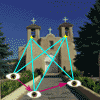## Imaging (Measurement) Model

Although estimating the parameters of the motion model is the ultimate goal of this project, the real difficulty lies in the non-linear transformation that occurs in the measurement process. A simplistic model of the imaging process, ignoring higher order lens effects, was used. Assuming a point in a camera centered three dimensional frame of reference, the projection onto the image plane may be modeled as: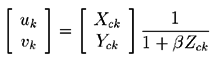where the inverse of the lens focal length,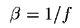, is used.

This model was recently proposed for use in this problem by Szeliski and Kang and also used by Azarbayejani et.al.. Geometrically, it is identical to the more common formulation :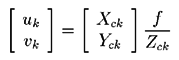The difference between the two is a change in the Z origin (from the POV in the traditional formulation to the image plane in the new one) and the calculation of the inverse focal length. The argument in favor of this formulation is that the traditional approach irrepairably couples the Zc estimate and the focal length estimate, making f merely a scaling factor. In addition, moving the origin makes the transformation stabler numerically at (artificially) long focal lengths (such as orthographic projection, where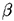= 0 ).

The "feature points" being tracked in the images have a globally referenced three dimensional location (often referred to as their "structure") that we may estimate given measurements from multiple camera locations.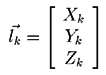Given this globally referenced location of a point, its location in the camera centered frame of reference is dependent on the camera location vector T and the camera orientation matrix R. The traditional geometry transformation equation for this mapping may be modified to support the proposed imaging geometry, giving: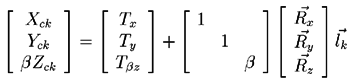The perceived depth (Zc) and camera translation in Z (Tz) are not calculated directly. Instead, BZc and Tzb are estimated, avoiding numerical instability in the case of long focal lengths.

I should point out that parts of the solution to the inverse imaging transformation may only be solved in a relative manner. The camera rotation and, the inverse focal length, may be determined absolutely, but the camera translation and the location of the feature points are in terms of an arbitrary spatial scale factor. We are all familiar with this lack of inherent scale in an image - we look for an object of known size to provide a measure of this scale factor. Similarly, this may be addressed in this problem by fixing the value of one of the relative parameters In the literature, various parameters are chosen: a component of the translational camera motion, or the location of a particular feature point.

The output of the imaging equation is a vector for each feature point. When discussing this problem, however, measurement components corresponding to a set of feature points are combined into a single measurement vector :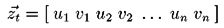The measurements which we can make are the location of the feature points in the camera image plane at different points in time/space. Combining the previous transformations gives us the following measurement equations :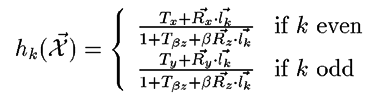Although nonlinear, these equations are relatively well behaved and differentiable.

The state vector of the model represents the position of the camera, its lens parameters, and the location of all the feature points being used. The state vector I chose to use is :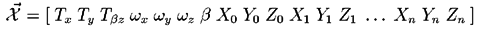More to come here...

Jump back to my NMM index page